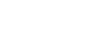# Pipe diameter and mean fluid flow velocity### How to calculate the pipe diameter and mean fluid flow velocity if flow is known?

The velocity of fluid flow is calculated as the ratio between the flow of fluid and the cross-sectional area of ​​the tube. The magnitude calculated in this way is the mean value of the fluid velocity. The actual velocity of the fluid in the pipe is not uniform across the cross-sectional area. The velocity of fluid flow is greatest in the axis of the pipeline, and is equal to zero at the point of contact between the fluid and the pipe wall. The mean velocity of fluid flow can be calculated as follows:In the case where the desired mean velocity of the fluid flow is known as well as the required volume flow through the pipe, the inner diameter of the pipe can be calculated according to the following:where is: D - internal pipe diameter; q - volumetric flow rate; v - velocity; A - pipe cross section area.

When, instead of the volume flow, the mass flow rate and the desired fluid flow rate are known, the internal diameter of the pipe can be determined as follows:where is: D - internal pipe diameter; w - mass flow rate; ρ - fluid density; v - velocity.

On the right side you can find three examples showing how you can calculate the pipe diameter if fluid flow and flow rate are known.

### Get Pipe flow calculator

Pipe diameter calculator allows you to calculate pipe diameter for known fluid flow and flow rate, as well as stream speed for known pipe flow and diameter. The calculator also allows conversion between volume and mass flow. With the help of a calculator you can calculate the volume flow of an ideal gas at different pressures and temperatures.

### Example no. 1

Task: Calculate the pipe diameter if the water flow velocity is 1 m/s and the flow rate is 5 lit/min

Solution: Pipe diameter is 10.3 mm

### Example no. 2

Task: Calculate the airflow velocity if the inner diameter of the pipe is 50 mm and the flow rate is 100 m3/h at a pressure of 3 bar absolute and a temperature of 15 °C

Solution: Flow velocity is 14.15 m/s

### Example no. 3

Task: Calculate the diameter of the carbon dioxide pipe with a flow rate of 10 kg/h and a velocity 2 m/s at a pressure of 7 bar absolute and a temperature of 20 °C.

Solution: Pipe diameter is 11.8 mm

### What is this?

The examples presented and their solutions are intended to present the possibility of calculator application in solving fluid flow problems in pipes.

Each example was solved using a calculator and the example solution shown was obtained by simply entering known quantities into the calculator, selecting units of measure and type of calculation.